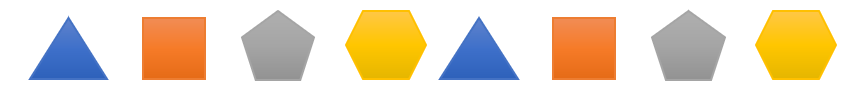Mathematics
Easy

Question

# Nancy is making a repeating pattern for the rule "Triangle, Square, Pentagon, and Hexagon."Which shape will be in the 49th shape in the pattern?

## Triangle    Square    Pentagon    HexagonHint:

## The correct answer is: Triangle

### The given pattern in words is Triangle, Square, Pentagon and Hexagon, Triangle, Square, PentagonWe are asked to find the shape at the 49th place.The rule used to write the pattern is “ Triangle, Square, Pentagon and Hexagon”.Four shapes are repeating. And, the fourth shape is a hexagon.So, all multiples of four have hexagon in their position.We have to find the shape at 49th place. It is not a multiple of 4.The number closest to 49 which is a multiple of 4 is 48. So, at 48th position there will be a hexagon.49 is one place after 48. The shape which will come one place after hexagon is a triangle.So, the shape at the 49th place is a triangle.

While solving such questions, we have to be careful about the order of the sequence.

### Related Questions to study#### With Turito Foundation.#### Get an Expert Advice From Turito.